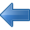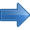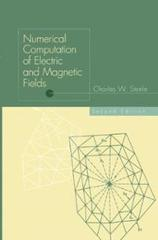Electrostatic Applications Books by Topic Author IndexPrevious Computer and mathematical models Next# Numerical Computation of Electric and Magnetic Fields, 2nd ed.

## Charles W. Steele

1996

xv + 260 pages

This new edition reports on the advances in the state-of-the-art of field computation since the book's first edition in 1987. The book provides:

Contents

## List of Contents

Field Properties
Introduction
Maxwell's Equations in the Dynamic, Quasi-Static, and Static Cases
Polarization and Magnetization
Laws for Static Fields in Unbounded Regions
Integral Representations for Quasi-Static Fields Using the Helmholtz Theorem
Equivalent Configurations
Steady-State Dynamic Problems and Phasor Field Representations
Continuity Conditions of Fields at a Medium Discontinuity References
Problem Definition
Introduction
Field Problem Domains, Source Problem Domains, Interior Problems, and Exterior Problems
Is the Problem Static, Quasi-Static, or Dynamic?
What Field Is To Be Computed?
Is the Problem Two-Dimensional or Three-Dimensional?
The Medium
Boundary Conditions and Uniqueness of Solutions
References
Linear Spaces in Field Computations
Introduction
Basis Functions
Shape Functions
Finite Elements and Shape Functions of Global Coordinates in Two-Dimensional Problem Domains
Isoparametric Shape Functions in Two Dimensions
Finite Elements and Shape Functions of Global Coordinates in Three-Dimensional Problem Domains
References
Projection Methods in Field Computations
Introduction
Special Spaces in Field Computations
Operators in Field Calculations
Approaches Used in Obtaining Approximate Solutions to Field Problems
Finite Element Method for Interior Problems
Integral Equation Method
Projection Methods
Orthogonal Projection Methods References
Finite Element Method for Interior Problems
Introduction
Formulation of Finite Element Method for Interior Problems
Computation of Linear System for Finite Element Method
Sample Problem
References
Finite Element Method for Exterior Problems
Introduction
McDonald-Wexler Algorithm
Silvester et al. Algorithm
Mapping Algorithms
References
Automatic and Adaptive Mesh Generation
Introduction
Preliminary Mesh Generation
Delaunay Tesselation
An Algorithm for Local and Global Error Estimation
Mesh Refinement Algorithm References
Integral Equation Method
Introduction
Linear and Uniform Media in Continuity Subdomains
Saturable, Nonlinear, and Nonuniform Media in Continuity Subdomains
Numerical Solution of Integral Equations General Approach
Finite Elements and Basis Functions Used in the Integral Equation Method
Integral Equation Numerical Solution by the Collocation Method
Integral Equation Numerical Solution by the Galerkin Method
Numerical Integration
Sample Problem
References
Static Magnetic Problem
Introduction
Interior Static Field Problems
Exterior Static Problems Approximated by Interior Problems
Exterior Magnetic Field Static Problem
Static Magnetic Field in a Saturable Medium
References
Eddy Current Problem
Introduction
Commonly Used Basic Formulations for the Eddy Current Problem
Two-Dimensional Eddy Current Problem
Three-Dimensional Steady-State Eddy Current Problem
Transient Eddy Current Problem
References
Derivation of the Helmholtz Theorem
Properties of the Magnetic Vector Potential, A Proof Regarding Split of Quadrangle into Two Triangles
Derivation of Formulations Used in the Cendes-Shenton Adaptive Mesh Algorithm
Integral Expressions for Scalar Potential from Green' s Theorem